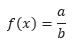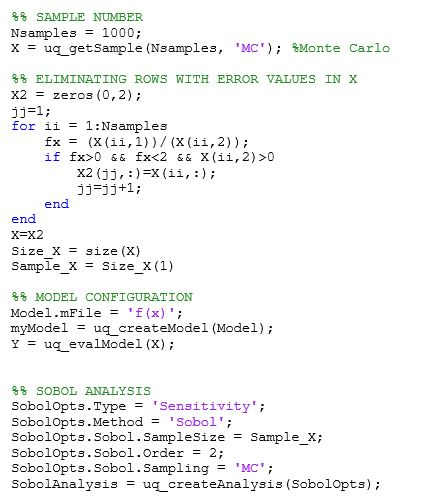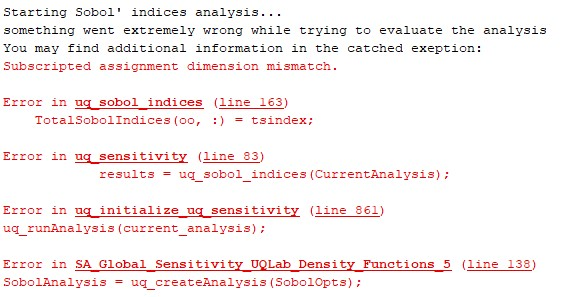# Redefine the size of the sampling vector obtained by the uq_getSample function

Hello UQWorld!

I have the following question. I am conducting a global sensitivity analysis (Sobol using Monte Carlo sampling) and was wondering if it is possible to redefine the size of the sampling vector obtained by the uq_getSample function or, alternatively, set a constraint between two parameters involved in the sensitivity analysis without changing their probabilistic density functions?

The model it’s a simple equation that has two parameters:Parameter (a) needs to be modeled by a log-normal distribution and parameter (b) by a Normal distribution.

In order to guarantee the convergence of the model, it is required that the values of the function f(x) must be between ]0, 2[ i.e. 0 < f(x) < 2. This should always be accomplished, otherwise the results may diverge.

For the performed analysis I can’t redefine the density functions of the parameters like it was explained in the forum Topic: Variable-dependent constrain on parameters, because both parameters have different type of probability density functions.

I’ve tried to solve this problem by eliminating sampling values that do not meet the given condition, as it’s shown below.But this solution generates an error on dimension mismatch after running the Sobol’ analysis:The model fails when it tries to calculate the total Sobol’ indices on the uq_sobol_indices core, because tsindex is a NaN vector. This occurs since the AllValues vector in the uq_sobol_indices core includes NaN values.

Since the uq_evalModel core does not show its code, it is assumed that the modified sample has not been evaluated, but rather the initial sampling.

Is there a way to evaluate a bounded sample or model the dependency between the parameters without changing the distribution functions?

I will be grateful for any recommendation,

Thanks,

Isabelle

Dear Isabelle

There is an issue with your problem formulation. If a ratio of two parameters a/b must be bounded between 0 and 2 so that your model converge (i.e the physics makes sense) you cannot choose independently distributions on a and b that don’t follow this condition.
Moreover having the inverse of a Gaussian in an expression is usually not good because of the singularity around 0: the resulting distribution has weird properties (e.g no variance).

One way to approach your problem would be to assign distributions to a and to the ratio, using a bounded distribution for the latter.

Finally note that as soon as you have dependence between parameters the standard Sobol indices resulting from variance décomposition cannot be used. You can find more information in the Sensitivity Manual.

Best regards
Bruno

Hi @Imfett , welcome to UQWorld!

Estimating Sobol’ indices by Monte Carlo (MC) simulation requires a specific set of sample points generated from your input. The scheme to generate this sample is similar to the one illustrated in this Wikipedia entry. In UQLab, you cannot generate your sample in advance and use it compute the Sobol’ indices; that is, your precomputed `X` and `Y` cannot be used.

Assuming that you have created INPUT and MODEL objects named `myInput` and `myModel`, here’s what happens behind the scene, when you run the Sobol’ analysis:

1. UQLab generates the required sample points from your INPUT object.
2. UQLab evaluates your model using the generated sample points to get the outputs.
3. UQLab computes the Sobol’ indices using one of the estimators given in the Sensitivity user manual Section 1.5.3.3.

By default, UQLab uses the currently selected (lastly created) INPUT and MODEL objects to do the above steps. That’s why you don’t need to specify `myInput` and `myModel` explicitly in `SobolOpts`.

The sample size you specified in `SobolOpts.Sobol.SampleSize` corresponds to the number of MC sample points used in the estimation.

Note that the total number of model evaluations to estimate the first-order and total order Sobol’ indices for each of your model parameters is N(M+2) where N is the `SampleSize` and M is the number of model parameters. More will be needed if you ask for higher-order Sobol’ indices. This number can be large, thus for expensive (to evaluate) computational model it might be a good idea to create a metamodel first (say, PCE).

Referring to your code, you should not expect by assigning `Sample_X` to `SobolOpts.Sobol.SampleSize`, UQLab will recognize that the inadmissible output values of your model have been filtered. This field is just to specify the number of MC sample size and in this case, the sample is less than the initial `NSamples` of 1'000 due to the filtering.

``````Model.mFile = 'f(x)';
myModel = uq_createModel(Model);
Y = uq_evalModel(X);
``````

Which `f(x)` are you referring to here? Is there really a MATLAB file named `f(x)` or is it the variable `fx` you defined inside the for loop above? If it’s the latter, then this is also problematic because you need an actual valid MATLAB function (either as m-file, function handle, or text string) to create a MODEL object. If `f(x)` is an already precomputed variable, it wouldn’t make sense to evaluate it using `uq_evalModel` at X.

Once again though, you should first resolve the problem conceptually as suggested by Bruno to either make your inputs independent and continue using Sobol’ indices or use alternative dependent sensitivity measures.

Dear Bruno and Damar,

Thank you for answering soon my concern.

Answering Damar question, there is a MATLAB file named f(x) and has not been computed before. In fact, the analysis runs without problem if the “new code” (where the sample is bounded) is not executed and if b value does not take the 0 value, but the Sobol’ indices diverge.

I will try to solve the problem conceptually and then evaluate assign distributions to a and to the ratio using a bounded distribution or use alternative sensivity measures.

Thank you very much for your answers and explaining in detail how different UQLab cores works. I understand know.

Best regards,

Isabelle

Great! Let us know if you manage to solve your problem or have further questions.

Yes, of course. Otherwise it should have thrown an error when the model was created. I missed that. Before, I was just not sure and a bit curious if `f(x)` having parentheses was a valid filename for a MATLAB function; apparently it is. Which MATLAB version are you using? on which operating system? Thanks!

Dear Damar,

Thank you for the interest. I have change the f(x) file name for fx to be sure that the filename is not the problem.

Iam using MATLAB version 9.3.0.713579 (R2017b) and my operating system is a Windows 10 Home (version 1903) x64 bits.

I’ll let you know if I have further questions, but with the answers you and Bruno gave me I undestand how to solve my problem. Thank you both for the support and your answers.

Thanks! and Best regards,

Isabelle